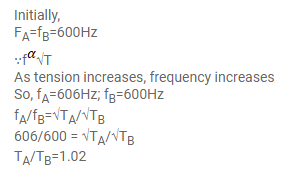# A piano wire A vibrates at a fundamental frequency ofQuestion:

A piano wire $A$ vibrates at a fundamental frequency of $600 \mathrm{~Hz}$. A second identical wire $B$ produces 6 beats per second with it when the tension in $A$ is slightly increased. Find the the ratio of the tension in $A$ to the tension in $B$.

Solution: